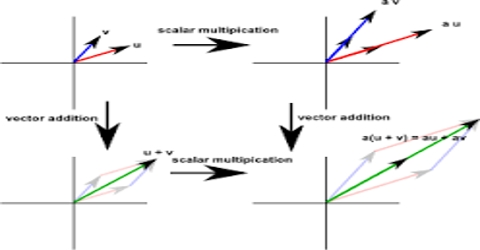# Vector Properties

Vector Properties

To describe a physical quantity indicative of direction is required along with magnitude. These physical quantities are vector quantities. If such quantifies of the universe are not expressed by magnitude and direction, then the expression of these quantities remain incomplete. Vector quantities follow or obey some rules; e.g.:

• Vector quantity has magnitude and direction.
• Two or more homogeneous vectors can be added. Different kind of vectors cannot be added.
• When two or more vectors are added, then the resultant vector is equal to the result of total action of the first two vectors.
• The vector product of two vectors is a vector quantity.
• The scalar product of two vectors is a scalar quantity.
• The direction of a vector is indicated by the proportion of the vector quantity and its magnitude.
• Vector quantity obeys the rules of addition and distribution.
• Vector quantity can be divided into components.
• The magnitude of the vector might be represented by absolute value signs around the vector symbol, or just the letter without the boldface.